CIS.PDI. Properties of the Definite Integral.
The important properties of the definite integral are described as the Sum Property, the Scalar Multiple Property, and the Addition Property.
These properties are usually connected to the integral's motivating interpretation as an area of a planar region.
Mapping diagrams can also play an important supporting role in visualizing the properties of the definite integral as well as their proofs
Suppose $D=[a,b]$,  is a closed and bounded (compact) interval,
$a \le c\le b$, and $P, Q : D \to \mathbb{R}$ are integrable over $[a,b]$.
 Sum Property Scalar Multiple Property Addition Property $\int_a^b P(x) + Q(x) \ dx = \int_a^b P(x)\ dx + \int_a^b Q(x) \ dx$ $\int_a^b \alpha P(x)\ dx = \alpha \int_a^b P(x)\ dx$ $\int_a^b P(x)\ dx = \int_a^c P(x)\ dx + \int_c^b P(x) \ dx$ Area-Graph Interpretation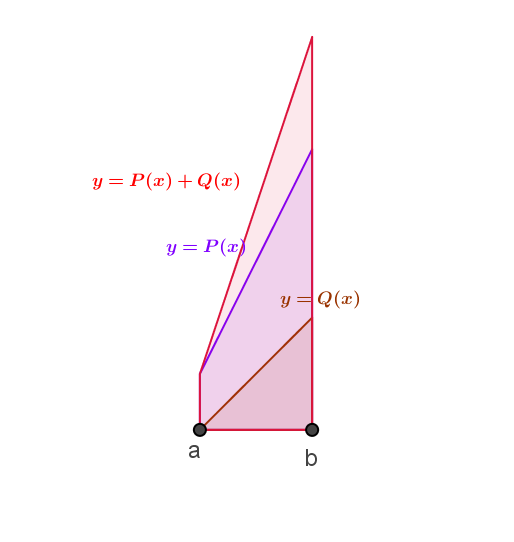The area interpretation visualizes the graphs of the functions, $P$and $Q$, with the graph of the function $P+Q$ on the same interval $[a,b]$ and the property is understood by recognizing the region determined by $P+Q$ as combining the regions determined by $P$ and $Q$ with the areas (definite integrals) being added.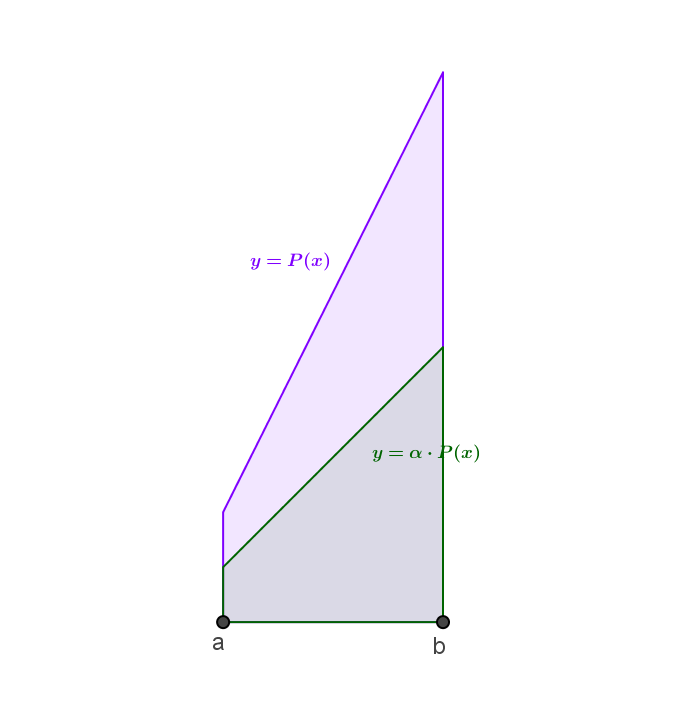The area interpretation visualizes the graphs of the functions, $P$ and $\alpha P$, with the graph of the function $\alpha P$ on the same interval $[a,b]$ and the property is understood by recognizing the region determined by $\alpha P$ as scaling  the region determined by $P$  the area (definite integral) being multiplied by $\alpha$.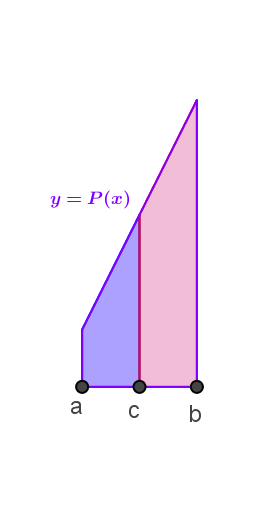The area interpretation visualizes the graph of the function, $P$, on the intervals $[a,b]$,$[a,c]$, and $[c,b]$ and the property is understood by recognizing the regions determined the region determined by $[a,b]$ as combining the regions determined by $[a,c]$ and $[c,b]$ with the areas (definite integrals) being added. Motion-Mapping Diagram Interpretation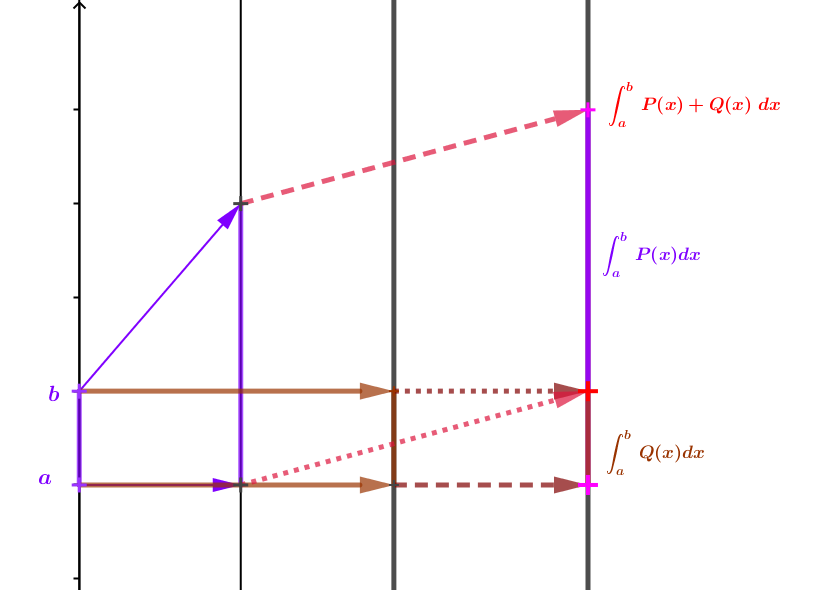The mapping diagram visualizes the integrals as the net change in position for an object moving over the time interval $[a,b]$ with velocities of $P,Q$, and $P+Q$. The result for the object moving with the velocity $P+Q$ (that adds the separate velocities) will add the net changes for objects traveling with the separate velocities.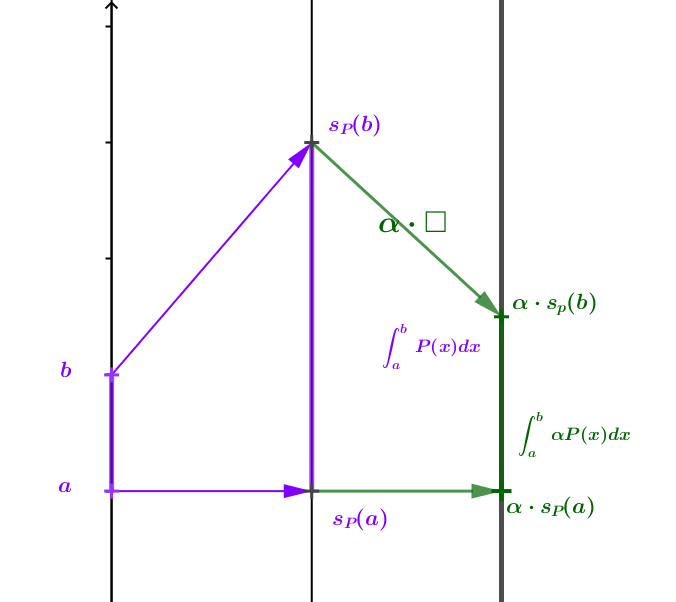The mapping diagram visualizes the integrals as the net change in position for an object moving over the time interval $[a,b]$ with velocities of $P$ and $\alpha P$. The result for the object moving with the velocity $\alpha P$ (that scales the original velocity) will multiply the net change for object traveling with the velocity $P$ by $\alpha$.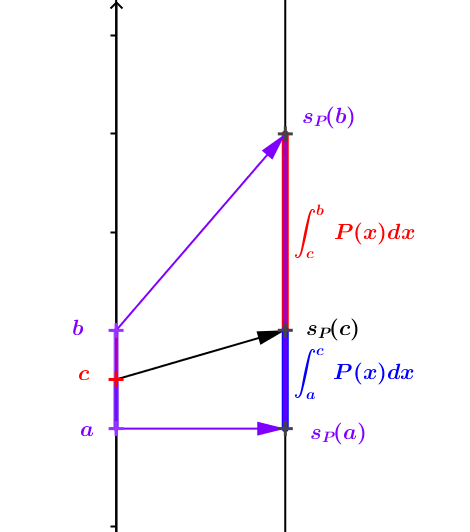The mapping diagram visualizes the integrals as the net change in position for an object moving over the time intervals $[a,b]$,$[a,c]$, and $[c,b]$ with velocity of $P$. The result for the object moving with the velocity $P$ over the interval $[a,b]$  will add the net changes for object traveling over the separate intervals.